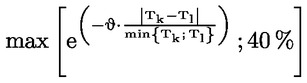In force

Version from: 01/01/2023
Amendments
Search within this legal act

### Article 325af - Intra bucket correlations for general interest rate risk

Article 325af

Intra bucket correlations for general interest rate risk

1.
Between two weighted sensitivities of general interest rate risk factors WSk and WSl within the same bucket, and with the same assigned maturity but corresponding to different curves, correlation ρkl shall be set at 99,90 %.
2.

Between two weighted sensitivities of general interest rate risk factors WSk and WSl within the same bucket, corresponding to the same curve, but having different maturities, correlation shall be set in accordance with the following formula:where:

 Tk (respectivelyTl) = the maturity that relates to the risk free rate;
 θ = 3 %
3.
Between two weighted sensitivities of general interest rate risk factors WSk and WSl within the same bucket, corresponding to different curves and having different maturities, the correlation ρkl shall be equal to the correlation parameter specified in paragraph 2, multiplied by 99,90 %.
4.
Between any given weighted sensitivity of general interest rate risk factors WSk and any given weighted sensitivity of inflation risk factors WSl, the correlation shall be set at 40 %.
5.
Between any given weighted sensitivity of cross-currency basis risk factors WSk and any given weighted sensitivity of general interest rate risk factors WSl, including another cross-currency basis risk factor, the correlation shall be set at 0 %.